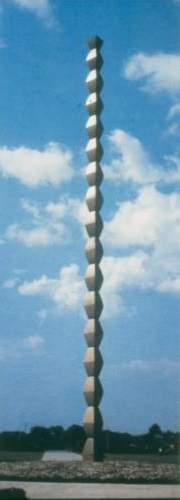Author: Dr. Allan B. Cruse, Professor Emeritus, Computer Science and Mathematics, University of San Francisco

## On rescaling rectangular images to fit a fixed-width webpage

 Assume we have a set of   n   rectangular images whose widths and heights (expressed in pixels) are, respectively,                         W1-by-H1,       W2-by-H2,       W3-by-H3,       ... ,       Wn-by-Hn. We would like to display these images side-by-side on a fixed-width webpage, but in cases where their heights may differ, or where the total of their widths does not match the width of our webpage's display-area, then some rescaling of these images will need to be performed, perhaps by using an image-editing tool such as the Microsoft Paint program or the Linux Gimp utility. A simple example of this situation occurs when we receive a pair of small-size photographs, sent to us via email from an iPhone, such as the two below -- one having landscape orientation, the other having portrait orientation:The dimensions of the left-hand photo are   320-by-240   whereas the dimensions of the right-hand photo are   240-by-320   . To determine the appropriate scaling factors   s1, s2, s3, ... , sn   and, simultaneously, the uniform pixel-height   u   for the   n   rescaled images, we can formulate a system of   n+1   linear equalities in these   n+1   unknowns:                                     H1*s1 = u                                     H2*s2 = u                                     H3*s3 = u                                           ...                                     Hn*sn = u                                     W1*s1 + W2*s2 + W3*s3 + ... + Wn*sn = D where the constant   D   denotes the horizontal display-width of our webpage, expressed in pixels. Here the first   n   equations express the condition that, after being rescaled, all the rectangles will have the same height   u   , while the final equation expresses the requirement that, after rescaling, the sum of these   n   image-widths will be equal to the webpage's display-width   D   . Using these equations it is straightforward to express the   n   scaling-factors   s1, s2, s3, ... , sn   in terms of the unknown uniform image-height   u   as follows:                         s1 = u/H1,         s2 = u/H2,         s3 = u/H3,         ... ,         sn = u/Hn and then substitute these expressions in the final equation:                         W1*(u/H1) + W2*(u/H2) + W3*(u/H3) + ... + Wn*(u/Hn)   =   D which can immediately be solved for   u  :                         u   =   D   /   [ (W1/H1) + (W2/H2) + (W3/H3) + ... + (Wn/Hn) ] For the special case of the preceeding two-image example, if we have   D = 700,   then the uniform image-height   u   will be   u = 336                 u   =   700 / [ (320/240) + (240/320) ]   =   700 / [ 4/3 + 3/4 ]   =   700 / [ 25/12 ]   =   336 The scaling-factor   s1 = 336/240 = 1.4   transforms the 320-by-240 landscape-format rectangle into a 448-by-336 image, while the scaling-factor   s2 = 336/360 = 1.05   transforms the 240-by-360 portrait-format rectangle into a 252-by-336 image, so that they fit neatly into our webpage display:The width-to-height fractions   W1/H1,   W2/H2,   W3/H3,   ... ,   Wn/Hn   are known as aspect ratios, and employing that terminology allows us to formulate our general mathematical result in a way that may be easily remembered:           When rescaling a set of rectangular images for side-by-side display, their uniform           image-height   u   should be equal to the webpage's display-width   D   divided by           the sum of the images' aspect ratios. We can illustrate this general rule with one further example involving a triple of rectangular images downloaded from the internet:Sizes of these three images are, respectively,   183-by-275,   297-by-250,   and   250-by-188 , so their aspect ratios, from left-to-right, are (approximately)   0.665,   1.188,   and   1.330,   with sum equal to   3.183.   Dividing display-width   D = 700   by this sum gives   u = 220   and the resulting rescaled equal-height images are now exhibited side-by-side below:## Exercises

1. Compute rescaled sizes for side-by-side display of these three images if page-width is 700 pixels:2. Compute rescaled sizes for these same three images if the latter two are shown one-above-the-other.

 NOTE: You can discover the dimensions (width and height) for each of these images           by moving your mouse-cursor over it and pressing the right-hand mouse-button.

Written on 28 FEB 2013; last revised on 22 APR 2013